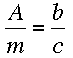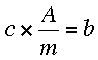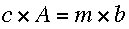SEARCH HOMEMath Central Quandaries & QueriesSolve for c A over m = b over c sorry I can't put them over each otherHi Steffane,

When I seeI want to eliminate the fractions so I first multiply both sides by c to getThere is still a fraction remaining so multiply both sides by m to getNow can you solve for c?
PennyMath Central is supported by the University of Regina and The Pacific Institute for the Mathematical Sciences.